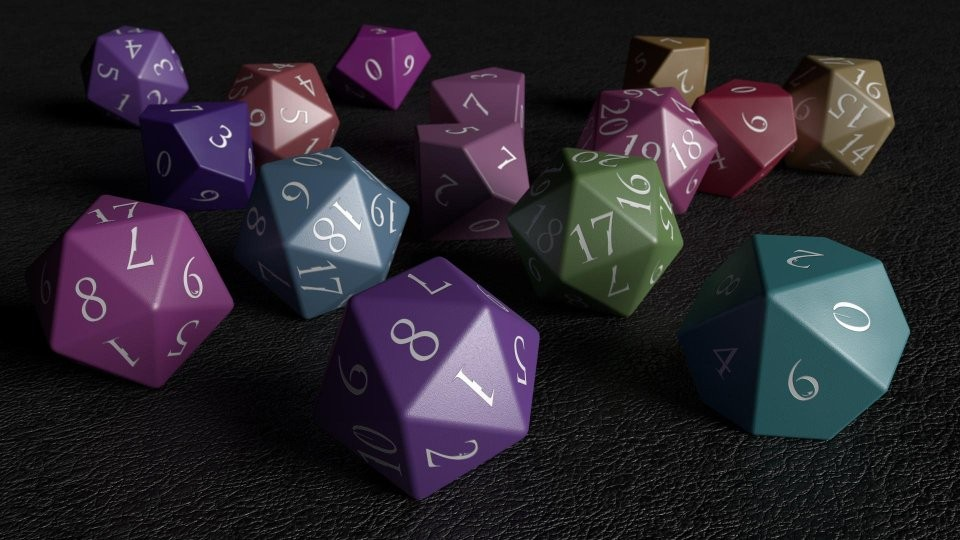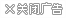# MisterB的扮演角色扮演者的技能369
108 人收藏
771 次下载
3109 次浏览
1.0

• 详细介绍
• 更新记录
• 玩家评论

[技能帽]

SkillsCaps设置为255

SkilFormulaCaps设置为155

[LevelSkillExpMults]

Level Skill Exp Multi是第一个调整您最终收到的体验的修饰符。这部分决定了我脑海中技能的固有难度。然而，由于炼金术魔药和食物调整，炼金术在这一点上并没有被削弱。

fAlchemy = 1.0

fAlteration = 0.85

fMarksman = 0.65

fBlock = 0.75

fConjuration = 0.7

fDestruction = 0.5

fEnchanting = 0.5

fHeavyArmor = 0.8

fIllusion = 0.75

fLightArmor = 0.9

fLockpicking = 1.0

fOneHanded = 0.75

fPickpocket = 0.8

fRestoration = 0.75

fSmithing = 1.0

fSneak = 1.0

fSpeechcraft = 1.0

fTwoHanded = 0.7

1 = 0.70

10 = 0.75

20 = 0.80

30 = 0.85

40 = 0.90

50 = 0.95

60 = 1.00

70 = 1.10

80 = 1.20

100 = 0.5

“第三个”和“最终”修改器根据基本技能级别设置附加乘数，每个技能独立。

1 = 1.00

10 = 0.90

20 = 0.80

30 = 0.70

40 = 0.60

50 = 0.50

60 = 0.60

70 = 0.70

100 = 0.50

[PerksAtLevelUp]

＃设置每个级别获得的额外津贴数量。如果未指定特定级别，则使用最接近的较低级别设置。这是该系统的主要变化。你在2级获得3个额外津贴，然后放慢速度，这样你在10级时最终获得10个额外津贴。

NOVICE TRAINING

2 = 3;我喜欢在第2级思考，你正在设定你的概念。这就是为什么你获得3个额外津贴来设置你的初始训练。

3 = 0.875

10 = 2;

11 = 0.90

20 = 2

21 = 0.80

30 = 2

31 = 0.70

40 = 2

41 = 0.60

50 = 2

51 = 0.50

60 = 2

61 = 0.4

70 = 2

71 = 0.3

[达到等级的能力点]

Health，Stamina和Magicka具有分层的进展水平。你的等级越高，得到的“越好”。因此，水平有更大的进步感和力量。像往常一样，我已经玩了50级，这是事情开始放缓的等级。越来越难以达到新的掌握水平，收益递减。你可以看到这反映在上面的图表中，显示了天际的5个掌握水平如何转化为水平。

1 = 8

10 = 9

20 = 10

30 = 11

40 = 12

50 = 11

60 = 10

70 = 8

[CarryWeightAtHealthLevelUp]

＃设置每个健康级别获得的携带重量的数量。如果未指定特定级别，则使用最接近的较低级别设置。

2 = 2

20 = 4

40 = 6

50 = 2

60 = 0

[CarryWeightAtStaminaLevelUp]

＃设置每个耐力级别获得的携带重量数量。如果未指定特定级别，则使用最接近的较低级别设置。

1 = 4

20 = 6

40 = 8

50 = 4

60 = 2

70 = 0

Vokrii  - 天际的简约特权

————————————————————————————————————————————

This uncapper has been designed for more of a slower roleplay style of game, which I have designed around several mods. But it would work with any set up where you want a more slower paced game. So let's break down the set up one piece at a time. I'm not very good at explaining things but I'll try my best.

[Skill Caps]
SkillsCaps are set at 255
SkilFormulaCaps are set at 155
Honestly these are set at what most uncappers I've seen are set up. Since I am unsure how to set these personally.

[LevelSkillExpMults]
The Level Skill Exp Multi is the 1st of thee modifiers that adjust your Experience which you end up receiving. This part decides the inherent difficulty of the skill in my mind. However Alchemy has not been nerfed at this point because of the mod Alchemy Potions and Food Adjustments.

fAlchemy=1.0
fAlteration=0.85
fMarksman=0.65
fBlock=0.75
fConjuration=0.7
fDestruction=0.5
fEnchanting=0.5
fHeavyArmor=0.8
fIllusion=0.75
fLightArmor=0.9
fLockpicking=1.0
fOneHanded=0.75
fPickpocket=0.8
fRestoration=0.75
fSmithing=1.0
fSneak=1.0
fSpeechcraft=1.0
fTwoHanded=0.7

The Second Part indicates how quickly people level depending on their experience level. The higher your level the quicker you level up in this case. This helps offset  the the difficulty of the actual skill.

1=0.70
10=0.75
20=0.80
30=0.85
40=0.90
50=0.95
60=1.00
70=1.10
80=1.20
100=0.5

The Third and Final modifier sets an additional multiplier depending on the base skill level, independently for each skill.

1=1.00
10=0.90
20=0.80
30=0.70
40=0.60
50=0.50
60=0.60
70=0.70
100=0.50

[PerksAtLevelUp]
#Set the number of perks gained at each level up. If a specific level is not specified then the closest lower level setting is used. This is the major change up of this system. You gain three perks at level 2, and then the perks are slowed down so you do end up getting 10 perks by the time you are level 10.

Each ten levels you gain 2 perk points, and slowly the perk gain slows down. So the higher your level gets the less you gain. For example from level 10 to 20, you gain only 9 perks instead of 10. For levels 20 to 30, you gain only 8 perks. However the bonus you gain every 10 levels should counter the eventual loss you gain as things become harder to learn.

Being able to simulate levels where you don't gain a perk point, I hope gives a sense of climbing the ladder of experience.

NOVICE TRAINING
2=3;  I like to think at Level 2, you are setting your concept in motion. Which is why you get 3 perks to set your initial training.
3=0.875

APPRENTICE TRAINING
10=2;
11=0.90

JOURNEYMAN TRAINING
20=2
21=0.80

30=2
31=0.70

EXPERT TRAINING
40=2
41=0.60

MASTER TRAINING
50=2
51=0.50
60=2
61=0.4
70=2
71=0.3

[Ability Points Gained at Level up]
Health, Stamina and Magicka have a tiered level of progression. Where the higher your level gets, the "better" you get. So that levels have a greater sense of progression and power. As usual I have played off that level 50 is the tier at which point things begin to slow down. Becoming harder and harder to reach new levels of mastery with diminishing returns. You can see this reflected in the chart above  showing how the 5 levels of mastery in Skyrim translates to level.

1=8
10=9
20=10
30=11
40=12
50=11
60=10
70=8

[CarryWeightAtHealthLevelUp]
#Set the number of carryweight gained at each health level up. If a specific level is not specified then the closest lower level setting is used.
2=2
20=4
40=6
50=2
60=0

[CarryWeightAtStaminaLevelUp]
#Set the number of carryweight gained at each stamina level up. If a specific level is not specified then the closest lower level setting is used.
1=4
20=6
40=8
50=4
60=2
70=0

Suggested Mods
Morningstar - Minimalistic Races  of Skyrim
Genders are Boringly Indistinct
Vokrii - Minimalistic Perks of Skyrim

1.版本号: 1.0   更新时间: 2019-09-11 22:35:24

• 感谢
• 支持
• 疑问
• 卖萌
• 关心
• 傲娇

作者精品### MisterB的扮演角色扮演者的技能

Mod大小：1.93 KB
上传时间：2019-09-11 22:14:34

### Mod简介：

暂无更多介绍

选择快速回复类型：
• 感谢
• 支持
• 疑问
• 卖萌
• 关心
• 傲娇

综合内容

游戏Mod

单机游戏

手机游戏